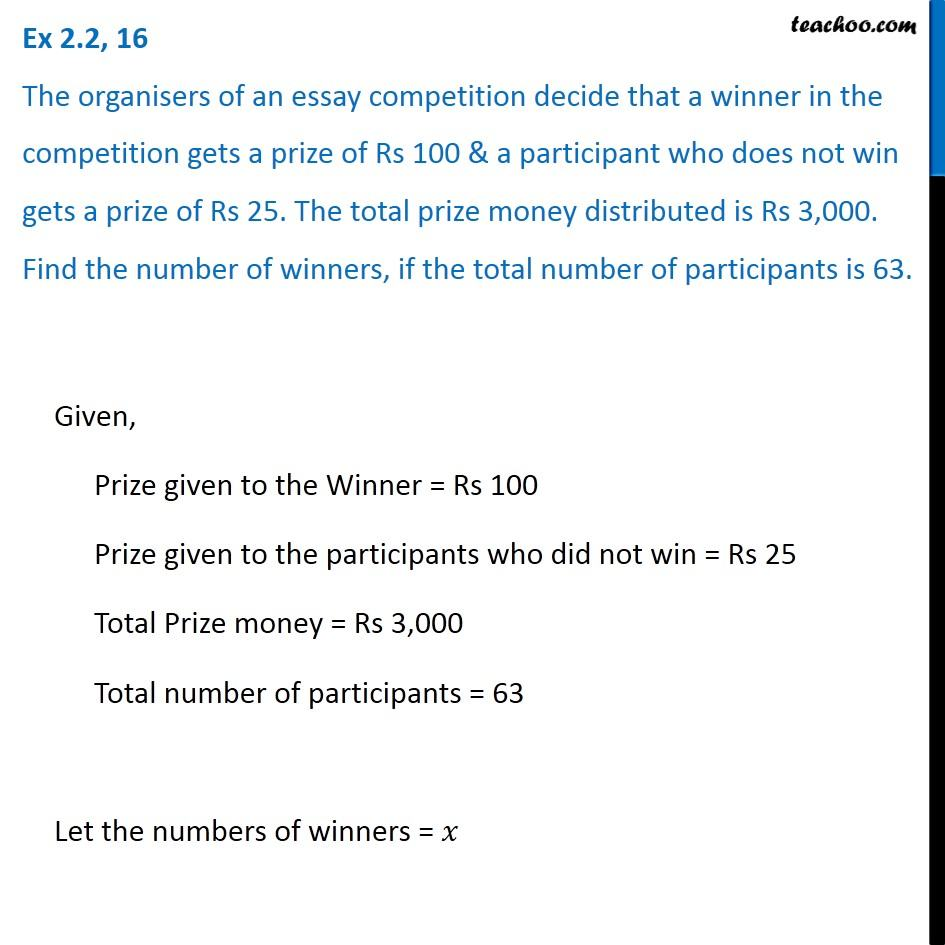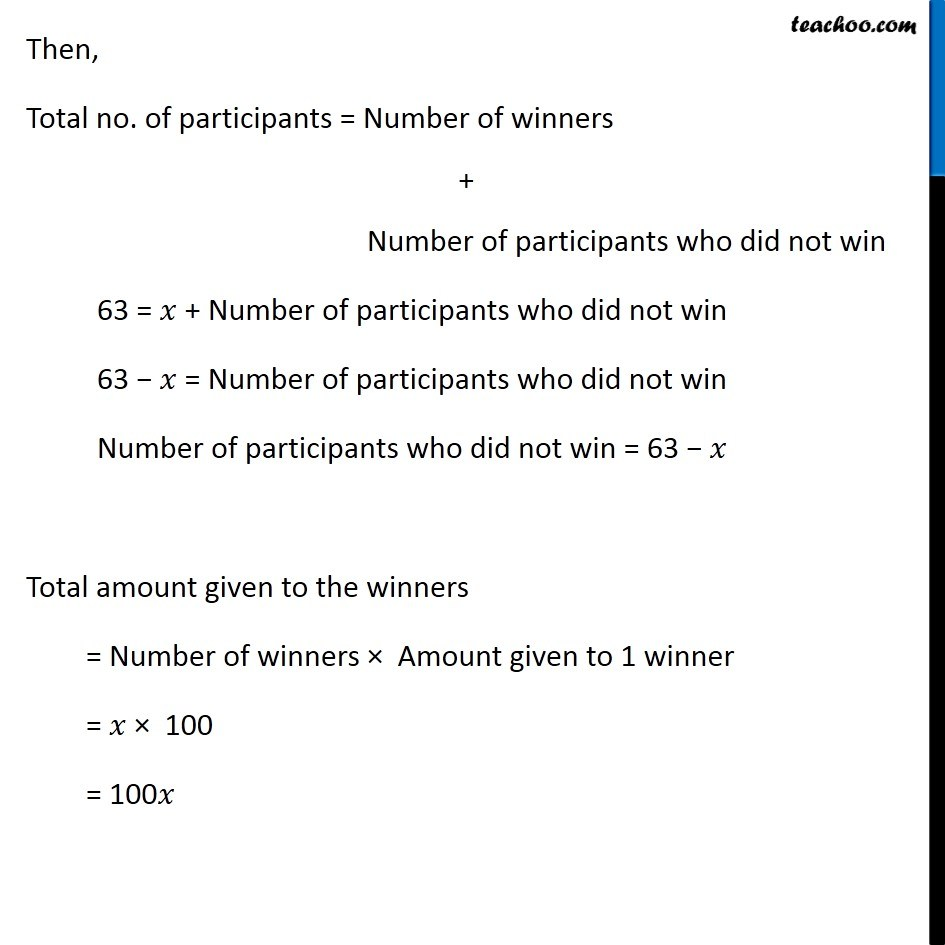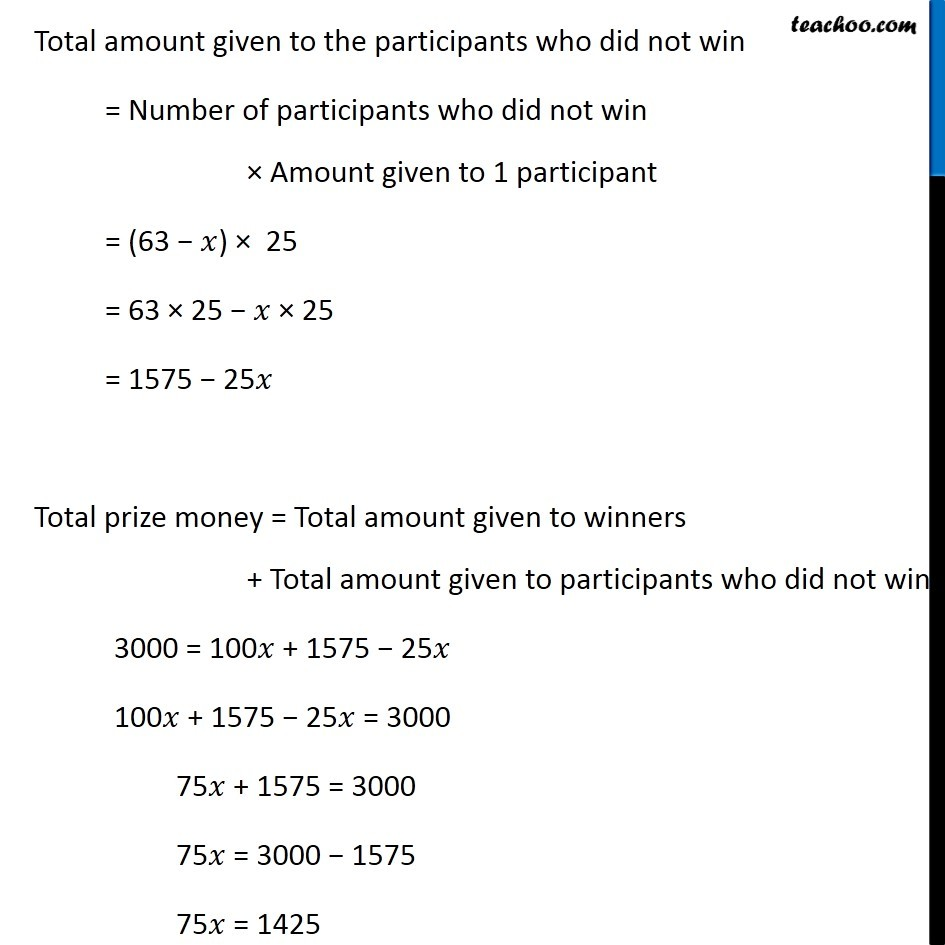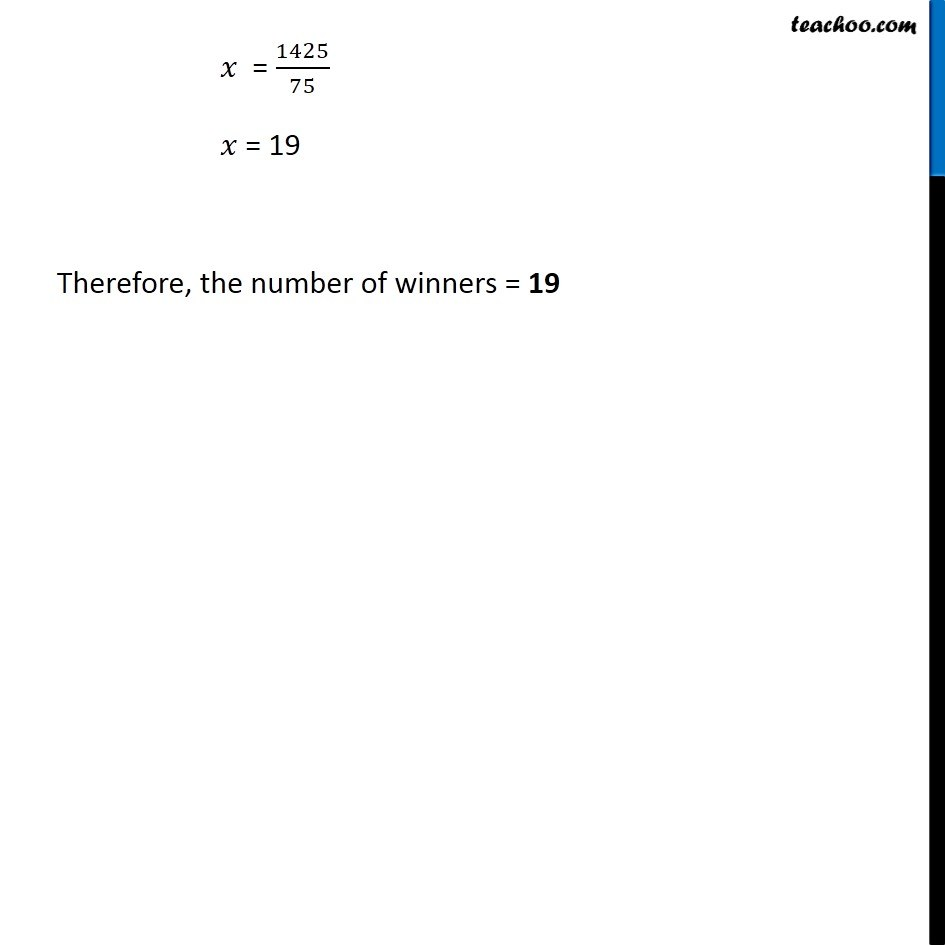Ex 2.2

Chapter 2 Class 8 Linear Equations in One Variable
Serial order wiseGet live Maths 1-on-1 Classs - Class 6 to 12

### Transcript

Ex 2.2, 16 The organisers of an essay competition decide that a winner in the competition gets a prize of Rs 100 & a participant who does not win gets a prize of Rs 25. The total prize money distributed is Rs 3,000. Find the number of winners, if the total number of participants is 63.Given, Prize given to the Winner = Rs 100 Prize given to the participants who did not win = Rs 25 Total Prize money = Rs 3,000 Total number of participants = 63 Let the numbers of winners = 𝑥 Then, Total no. of participants = Number of winners + Number of participants who did not win 63 = 𝑥 + Number of participants who did not win 63 − 𝑥 = Number of participants who did not win Number of participants who did not win = 63 − 𝑥 Total amount given to the winners = Number of winners × Amount given to 1 winner = 𝑥 × 100 = 100𝑥 Total amount given to the participants who did not win = Number of participants who did not win × Amount given to 1 participant = (63 − 𝑥) × 25 = 63 × 25 − 𝑥 × 25 = 1575 − 25𝑥 Total prize money = Total amount given to winners + Total amount given to participants who did not win 3000 = 100𝑥 + 1575 − 25𝑥 100𝑥 + 1575 − 25𝑥 = 3000 75𝑥 + 1575 = 3000 75𝑥 = 3000 − 1575 75𝑥 = 1425 𝑥 = 1425/75 𝑥 = 19 Therefore, the number of winners = 19